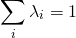# N-gram Language Models

Models that assign probabilities to sequences of words are called language models. An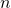gram is a sequence ofwords: a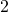-gram (or bigram) is a two-word sequence of words like “please turn”, “turn your”, or “your homework”, and a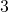-gram (or trigram) is a three-word sequence of words like “please turn your”, or “turn your homework”.

## Notation

To represent the probability of a particular random variable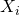taking on the value “the”, or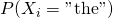, use the simplification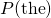.

A sequence of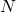words is represented either as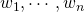or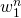.

## Probability of a Sequence

For the joint probability of each word in a sequence having a particular value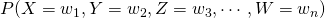use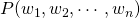.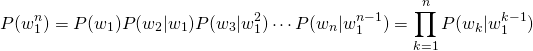The intuition of the-gram model is that instead of computing the probability of a word given its entire history, approximate the history by just the last few words.

## Bigram

The bigram model, for example, approximates the probability of a word given all the previous words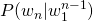by using only the conditional probability of the preceding word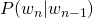.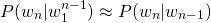This assumption that the probability of a word depends only on the previous word is also called a Markov assumption.

Thus, for a bigram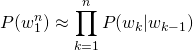## N-gram

The-gram (which looks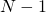words into the past) approximates the probability as follows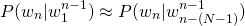## Parameter Estimation

Maximum Likelihood estimation is used to compute the parameters of an-gram model. This process involves getting counts from a corpus, and normalizing the counts so that they lie between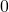and.

To compute a particular bigram probability of a word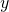given a previous word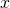, compute the count of the bigram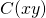and normalize by the sum of all the bigrams that share the same first word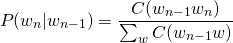The sum of all bigram counts that start with a given word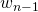must be equal to the unigram count for that word.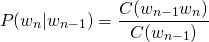For the general case of MLE-gram parameter estimation: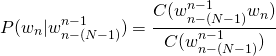## Perplexity

The perplexity (sometimes called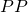for short) of a language model on a test set is the inverse probability of the test set, normalized by the number of words. The higher the conditional probability of the word sequence, the lower the perplexity. Consider the test set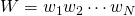.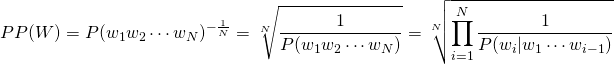For a bigram language model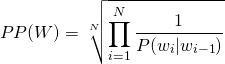The more the information the-gram gives about a word sequence, the lower the perplexity.

## Smoothing

Smoothing is a technique to prevent a language models from assigning zero probability to unseen events.

The unsmoothed Maximum Likelihood Estimate of the unigram probability of the word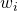is its count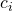normalized by the total number of word tokens.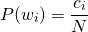Laplace (add-one) Smoothing technique adds a one to all the bigram counts. Since there are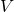words in the vocabulary and each one was incremented, the denominator needs to be adjusted to take into account the extraobservations.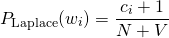Comparing the two equations above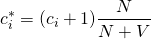The adjusted count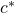describes how the smoothing affects the numerator.

The bigram probabilities follow the same technique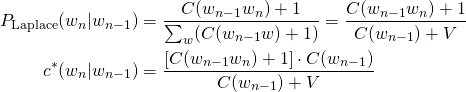An extension of the above technique is to add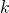instead of.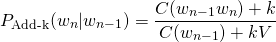## Interpolation

Interpolation is an approach to mix the probability estimates from all the-gram estimators. The trigram, bigram, and unigram counts are weighed and combined. An example of simple linear interpolation is given below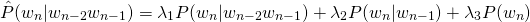such that